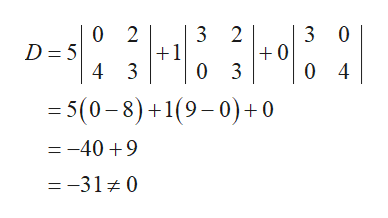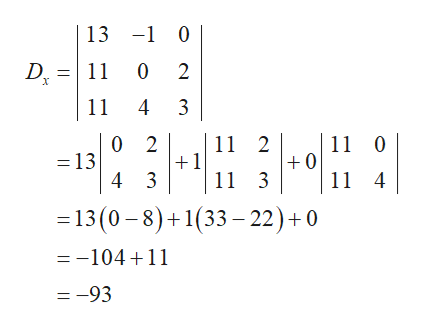# Use Cramer's rule to solve the following system of equations. If D = 0, use another method to solve the system.5x − y = 133x + 2z = 11 4y + 3z = 11

Question
27 views
 Use Cramer's rule to solve the following system of equations. If D = 0, use another method to solve the system.5x − y = 133x + 2z = 11 4y + 3z = 11
check_circle

Step 1

Consider the matrix as,

Step 2

First compute the value of D.help_outlineImage Transcriptionclose0 2 3 2 1 0 3 3 0 +0 0 4 D 5 4 3 =5(0-8)+1(9-0)+0 =-409 =-31 0 fullscreen
Step 3

Since the value of D is not equal to zero, use the Cramer’s rule and solve f...help_outlineImage Transcriptionclose13 -0 D 11 0 2 11 4 3 0 2 13 11 2 1 11 3 11 0 0 11 4 4 3 =13 (0-8)+1(33 22)+0 --104+11 =-93 fullscreen

### Want to see the full answer?

See Solution

#### Want to see this answer and more?

Solutions are written by subject experts who are available 24/7. Questions are typically answered within 1 hour.*

See Solution
*Response times may vary by subject and question.
Tagged in

### Other